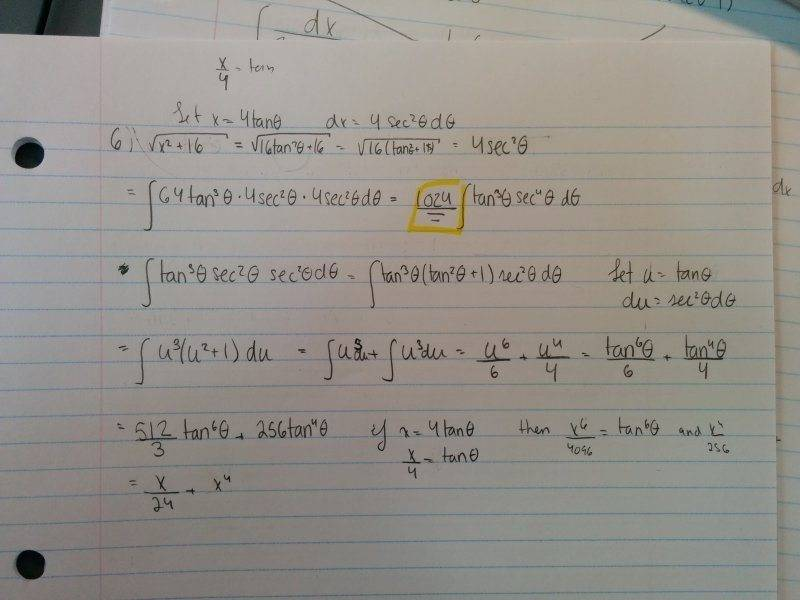# Integration Using Trigonometric Substitution Help Needed!

## Homework Statement

Integral of $$x^3\sqrt{x^2+16}dx$$
$$1/5(x^2+16)^{5/2} -16/3(x^2+16)^{1/2}+C$$

x=atanθ

## The Attempt at a SolutionMod note: The integral is ##\int x^3 \sqrt{x^2 + 16} dx##
The published answer is ##1/5(x^2+16)^{5/2} -16/3(x^2+16)^{1/2}+C##

To the OP: Using  (i.e., with a space between the $symbols) causes the browser to not render what's between the$ pairs. For inline LaTeX, you can use # # (no space) at the beginning and end.

#### Attachments

Last edited:

Please explain what you are trying to do

I'm trying to find the integral of the equation, but it doesn't give the same answer as the one I'm supposed to get, and I don't know what I'm doing wrong. I'm using trigonometric substitution which lets x=4sinΘ.

In the second step after you did the substitution, the whole things becomes,
4*((tan0^2)+1)^(1/2)=4sec0 and not 4sec0^2

•Airp
can you post the question? Like the integral you are trying to find the answer for?

I might be able to help you....I love integration...but I don't understand anything from the picture you posted

Mark44
Mentor
can you post the question? Like the integral you are trying to find the answer for?
See my previous post. It's fairly clear which integral the OP is trying to find.

Mark44
Mentor
No problem! I tried using Latex, but it doesn't work...It's #6
See my edit to post #1.

Thank You Mark! I'm still learning to use Latex... I changed it and it should appear ok, now!

Got it!!!

Ok, I'm currently trying, correcting the mistake I made with my sec^2. It looks good!

I can officially say that it works now! Thank you Faris! Such a "minor" mistake that really changed everything in the problem!

I might be able to help you....I love integration...but I don't understand anything from the picture you posted

He meant Question 6 from the picture

Your trig substitution is correct. When you square root, you should get 4sec(x) (I'll use x instead of theta for simplicity)
That will leave you with,
64tan3(x) * 4sec(x)

or simply
256tan3(x)*sec(x)

Break the tan3(x) up, you get
256tan2(x)*sec(x)*tan(x)

I wont do the rest for you but I'll give you a clue. It involves a trig identity and a u substitution

Thank you! It works now, my mistake was simply that I forgot to take the sqrt of sec^2

hey, if trigonometric substitution is not necessary, it is much easier to put x^2+16=t.......=>x^2=t-16.......dx=dt/2x.......x^3=x*x2.....
then integral is ##\int { x*(t-16)*\sqrt(t)*dt/2x} ## => ##\int {(t-16)\sqrt(t) dt/2} ## ............now expand normally...